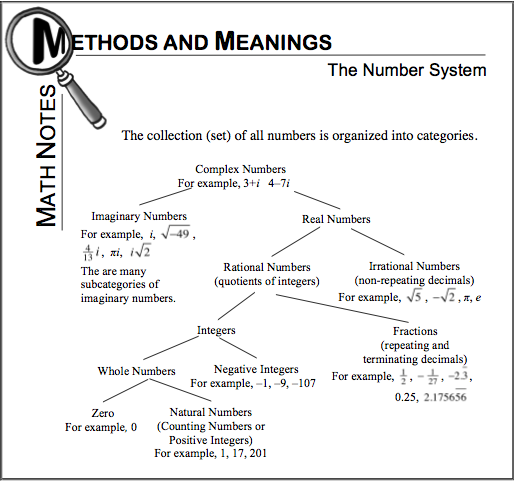Home > CCA > Chapter 10 > Lesson 10.2.5 > Problem10-82

10-82.

Identify each sum or product as a rational number or an irrational number. Homework Help ✎

Refer to the Math Notes box in this lesson (shown below) to help you with defining rational and irrational numbers.

1. $\sqrt{9}+\frac{5}{17}$

What is the square root of $9$? Can you simplify the answer to help you determine if it is irrational?

Rational

1. $\frac{2}{3}+\pi$

Is $\pi$ a rational number?

Irrational

1. $5·\sqrt{11}$

Use the same method as part (a).

Irrational

1. $\sqrt{12}·\sqrt{3}$